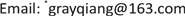1中国矿业大学信息与控制工程学院，江苏 徐州

2徐州市人工智能与大数据重点实验室，江苏 徐州1. 引言

2. 理论基础2.1. 秘密分享方案

Shamir的 ( k , n ) 门限秘密分享方案的基本思想是：首先，要把秘密S分割成 n 份影子信息；其次，分别将 n 份影子信息分发给 n 个参与者进行保管；最后，任意 k ( k ≤ n ) 份影子信息联合起来才能恢复出S。

f ( x ) = m 0 + m 1 x + m 2 x 2 + ⋯ + m k − 1 x k − 1 ， (1)

2.2. 直方图平移算法

Ni等人提出了一种基于直方图平移的可逆水印算法  ，其算法思想为：首先，绘出图像的灰度直方图，并找出其中的最大值点a和最小值点b，为方便讨论，不妨设 a < b ；其次，将图像中所有在 [ a + 1 , b − 1 ] 的灰度值加1，即将 [ a + 1 , b − 1 ] 的直方图向右平移一位，从而像素值为 a + 1 的像素点的个数变为0；再次，在嵌入水印时，若水印值为1，则像素值为a的灰度值加1，即为 a + 1 ；若水印值为0，则保持 a 的像素值不变；最后，提取水印信息时，顺序扫描图像，若像素灰度值为a，则提取的水印信息为0；若像素灰度值为 a + 1 ，则提取的水印信息为1  。若想恢复出原始宿主图像，只需把灰度值处在区间 [ a + 1 , b ] 的灰度值减1即可。

3. 新多图像水印算法3.1. 生成影子图像

3.2. 嵌入影子图像

3.3. 提取影子图像及恢复载体图像

w i j 1 ( x 1 ) = m 0 + m 1 x 1 + m 2 x 1 2 + ⋯ + m k − 1 x 1 k − 1 mod 255 w i j 2 ( x 2 ) = m 0 + m 1 x 2 + m 2 x 2 2 + ⋯ + m k − 1 x 2 k − 1 mod 255                                                                                                                                                                                                                                       ⋮                     w i j p ( x k ) = m 0 + m 1 x k + m 2 x k 2 + ⋯ + m k − 1 x k k − 1 mod 255 ； (2)

4. 实验验证

5. 算法分析

5.1. 透明性

PSNR = 10 lg 255 2 1 m × n ∑ i = 0 m − 1 ∑ j = 0 n − 1 ( X ( i , j ) − X W ( i , j ) ) 2 (3)

5.2. 鲁棒性

NC = ∑ i = 1 m ∑ j = 1 n W ( i , j ) W ′ ( i , j ) ∑ i = 1 m ∑ j = 1 n W 2 ( i , j ) (4)

5.3. 安全性

5.4. 嵌入容量

5.5. 算法执行效率

T ( n ) = O ( ( n − 1 ) + size + ∑ i ∈ U ( peak _ point , zero _ point ) h ( i ) + 1 2 h ( peak _ point ) ) (5)

6. 结论